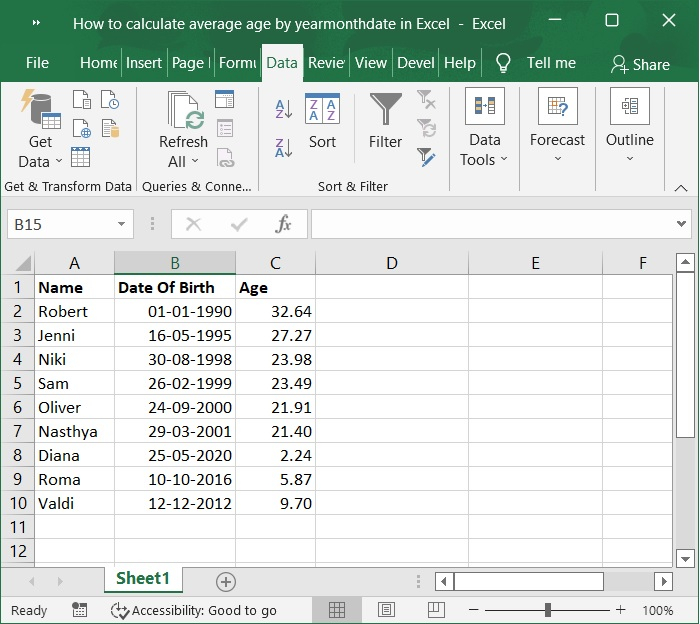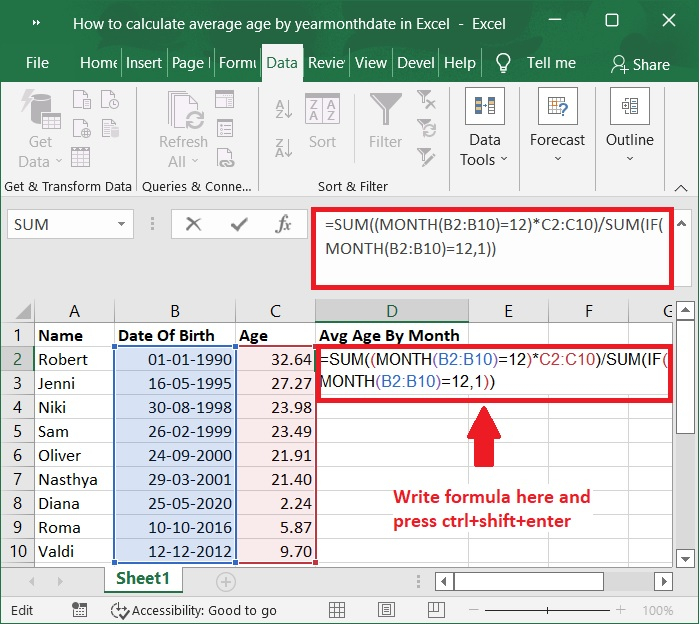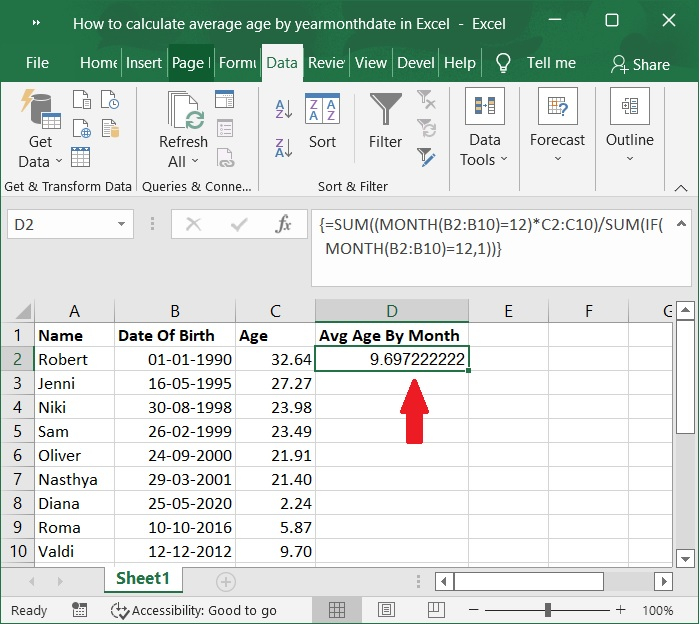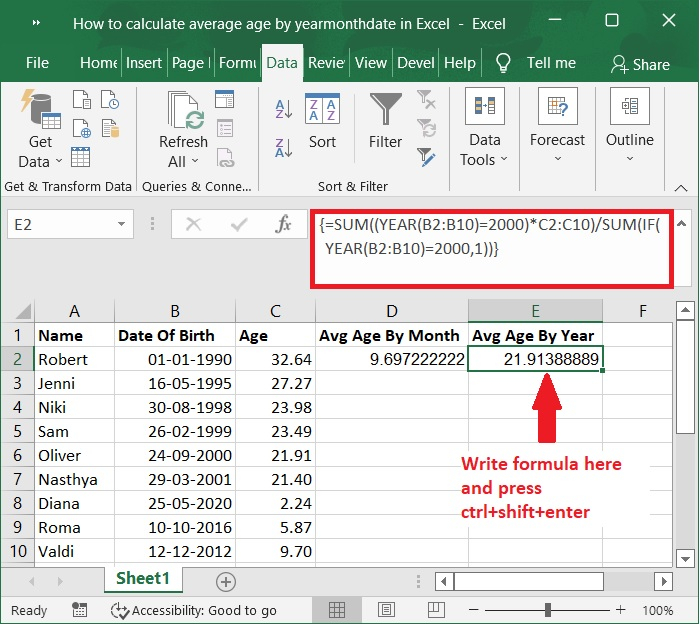# How to calculate average age by year/month/date in Excel?

If, for instance, you have a list of employee names in Excel along with their birthdays and ages, how would you go about calculating the average age for a specific month or year? And what happens if you want to calculate the average up to a certain date in Excel? In this tutorial, you will learn various Excel tips and methods that will make the problem easier to solve.

## Calculating the Average Age by Month with Formulas in Excel

Let’s understand step by step with an example.

### Step 1

For instance, you have a table that looks like the one shown in the accompanying screen shot for the same. Excel's array formulae make it possible to easily get the average age of a population based on either the year or the month.### Step 2

Now, choose a blank cell next to the table, like Cell D2, type the following formula −

=SUM((MONTH(B2:B10)=12)*C2:C10)/SUM(IF(MONTH(B2:B10)=12,1))


Then, press the Ctrl, Shift, and Enter keys at the same time to get the correct result. Please refer to the below screenshot for the same.Important note − In the above formula, the B2:B10 range represents the Date of Birth column, and the C2:C10 range represents the Age column. The number 12 represents the specified month "December" that you will average by; however, you are free to adjust these values to better suit your purposes.

### Step 3

Now, the calculated average age by month value is displayed in the cell D2. Below is the screenshot for the same.## Calculating the Average age by Year using formula in Excel

Choose a cell within the table that is blank, such as Cell E2, and type the following formula

=SUM((YEAR(B2:B10)=2000)*C2:C10)/SUM(IF(YEAR(B2:B10)=2000,1))


After that, press the Ctrl key together with the Shift key and the Enter key at the same time to get the correct results. Now, the calculated average age by year value is displayed in the cell E2. Below is the screenshot for the same.Important Note − In this method, the B2:B10 range represents the Date of Birth column, and the C2:C10 range represents the Age column. The year 2000 is the year you want to use as the benchmark for the average, although you are free to adjust these values to better suit your purposes.

## Conclusion

In this tutorial, we used a simple example to demonstrate how you can calculate the average age by year or month by using formulas in Excel.

Updated on: 03-Feb-2023

597 Views